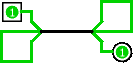# Splitters

When you work with multi-bit values, you will often want to route different bits in different directions. The Base library's splitter tool () allows you to accomplish this.

For example, suppose we want to build a circuit that computes the bitwise AND of the two nibbles of its eight-bit input (the upper four bits and the lower four bits). We will have an eight-bit value coming from the input pin, and we want to split that into two four-bit values. In the below circuit, we have used a splitter to accomplish this: The 8-bit input comes into the splitter (which resembles a bird's foot), which divides the 8 bits into two 4-bit values, which are then fed into the AND gate and from there to the output.In this example, the splitter happens to actually split an incoming value into multiple outgoing values. But splitters can also combine multiple values into a single value. In fact, they are non-directional: They can send values one way at one time and another way later, and they can even do both at the same time, as in the below example where a value travels eastward through the two splitters, then is routed back westward through them again, and then back eastward where it finally reaches its output.The key to understanding splitters is their attributes. In the following, the term split end refers to one of the multiple wires on one side, while the term combined end refers to the single wire on the other side.

• The Facing attribute tells where the split ends should be relative to the combined end.
• The Fan Out attribute specifies how many split ends there are.
• The Bit Width In attribute specifies the bit width of the combined end.
• The Bit x attribute says which split end corresponds to bit x of the combined end. If multiple bits correspond to the same split end, then their relative ordering will be the same as in the combined end. Logisim splitters cannot have a bit from the combined end correspond to multiple split ends.

Note that any change to the Fan Out or Bit Width In attributes will reset all Bit x attributes so that they will distribute the bits of the combined value as evenly as possible among the split ends.

Next: Wire colors.